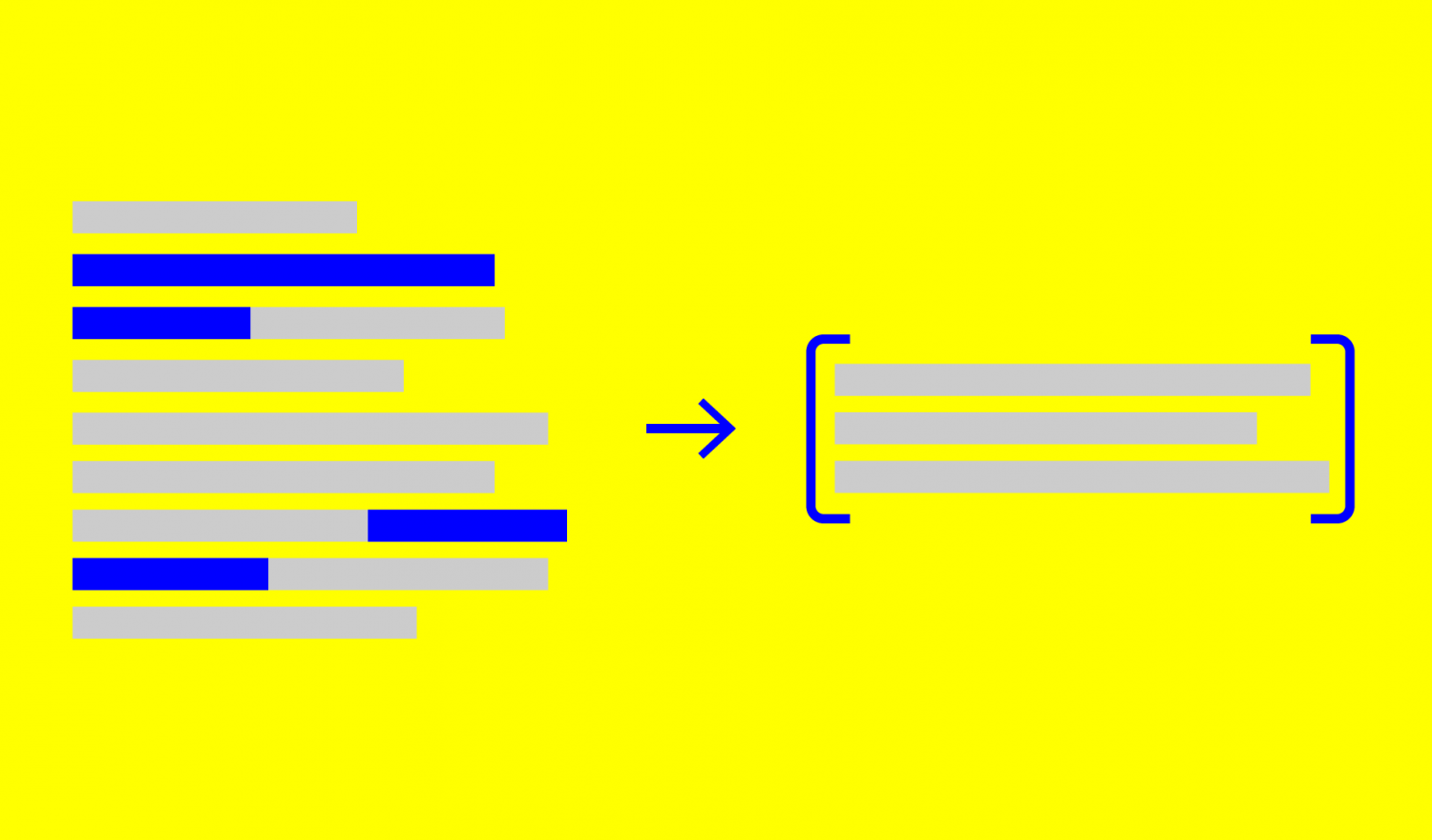Artificial Intelligence

# Summarizing Long Texts with Seq2Seq Neural Networks

Autor:in
Lesezeit
9 ​​min

This blog post describes my master thesis „Abstractive Summarization for Long Texts“. We’ve extended existing state-of-the-art  sequence-to-sequence (Seq2Seq) neural networks to process documents across content windows. By shifting the objective towards the learning of inter-window transitions, we circumvent the limitation of existing models which can summarize documents only up to a certain length.  With our windowing model we are able to process arbitrary long texts during inference. We evaluate on CNN/Dailymail  and WikiHow .

## The standard architecture for abstractive summarization in a nutshell

In a typical recurrent encoder-decoder Seq2Seq model used for abstractive summarization, a recurrent neural network (RNN) is trained to map an input sequence $$x_1, x_2,…,x_{T_x}$$ of word vectors to an output sequence $$y_1,…,y_{T_y}$$ which is not necessarily of the same length. Each of the $$x_1, x_2,…,x_{T_x}$$ words is coming from a fixed vocabulary $$\mathcal{V}$$ of size $$\left| \mathcal{V} \right|$$. The Seq2Seq model directly models the probability of the summary

$$\sum_{t=1}^{T_y}P(y_t|y_{t-1},y_{t-2},…,y_1,c_t; \theta)$$.

Given a set of $$N$$ training examples $$\{x^{(i)}, y^{(i)}\}_{i=1}^N$$, the usual training objective is to maximize the log-likelihood or equivalently minimize the negative log-likelihood of the training data,

$$\tilde{\theta}_{MLE} = argmax_{\theta}\{\mathcal{L}_{mle}\}$$

where

$$\mathcal{L}_{mle}(\theta) = \sum_{i=1}^N \sum_{t=1}^{T_y^{(i)}} \mathrm{log}\ P(y_t^{(i)}|y_{t-1}^{(i)},y_{t-2}^{(i)},…,y_1^{(i)},c_t^{(i)}; \theta)$$

A typical encoder variant is a bidirectional RNN, which yields an encoded representation $$h_j = [\overrightarrow{h}_j ; \overleftarrow{h}_j]$$ for each input symbol. The decoder is usually trained to act as a conditional language model which attempts to model the probability of the next target word conditioned on the input sequence and the target history. At each decoding step, it uses attention to focus on parts of the document relevant for the next prediction step. It forms an attention context vector  $$c_t = \sum_{j=1}^{T_x} \alpha_{tj}h_j \label{eq:convec}$$ with attention weights $$\alpha_{tj} =\frac{exp(e_{tj})}{\sum_{k=1}^{T_x} exp(e_{tk})}$$ and energies $$e_{tj} =a(s_{t-1}, h_j)$$, where $$s_{t-1}$$ is the previous decoder hidden state. In case of attention by Luong , the alignment is $$a(s_t, h_j) = s_t^T h_j$$.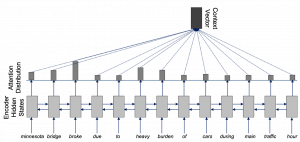Attention on bidirectional encoded hidden states forming the context vector

In attention by Luong, the decoder hidden state is updated by $$s_t = \tilde{f}(s_{t-1}, y_{t-1})$$. The conditional distribution of the next word over the vocabulary $$\mathcal{V}$$ is

$$P_{\mathcal{V}}(y_t|y_{t-1},y_{t-2},…,y_1,c_t) = \frac{\mathrm{exp}(l_{tk})}{\sum_{k=1}^{\mathcal{V}} \mathrm{exp}(l_{tk})}$$,

where the energy distribution is calculated by $$l_t = W^l \mathrm{tanh}([c_t;s_t]) + b_l$$.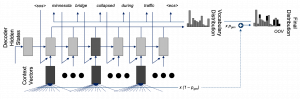Decoder with pointer-generator generates composed distribution to predict next word

The model is still constrained to model from a fixed vocabulary $$\mathcal{V}$$. If words are out-of-this-vocabulary, we cannot model them. To fix this, we use a pointer-generator network  which additionally allows copying form source. A generation probability $$p_{gen}$$ is calculated end-to-end at each decoding step $$t$$. It serves as a soft switch between sampling form $$P_V$$ and copying from the input sequence. The conditional probability for a word $$k$$ over the extended vocabulary $$\tilde{\mathcal{V}}$$ is

$$P_{\tilde{V}}(y_t=k|y_{t-1},y_{t-2},…,y_1,c_t) = p_{gen} P_V[k] + (1-p_{gen}) \sum_{j:x_j=k} \alpha_{tj}$$,

where $$\tilde{\mathcal{V}}$$ is defined as the union of $$\mathcal{V}$$ and all words in the source document.

## Limitation of the standard Seq2Seq model

When summarizing an article with the standard model, we have to truncate the document to the threshold $$T_x$$ that we trained on. For CNN/Dailymail, this is typically around $$400$$ words.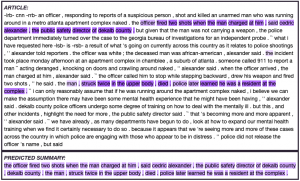Maximum attention visualization for standard model

An alternative would be to summarize longer text in chunks. However, this limits the coherence of the final summary as semantic information cannot flow between chunks. On top, finding the right chunking break points is non-trivial, as we have to ensure that at least locally semantic coherent phrases are within the same chunk.

## Solution: Windowed attention and inter-window transition learning

We extend the standard recurrent Seq2Seq model with pointer-generator to process text across content windows. Attention is performed only at the window-level. A decoder shared across all windows spanning over the respective document poses a link between attentive fragments as the decoder has the ability to preserve semantic information from previous windows. The main idea is to transform the learning objective to a local decision problem. The model slides only in forward direction and processes information from the current window based on the history accumulated over previous windows. By learning local transitions, during inference on long documents this capability can be exploited to process arbitrary long texts. The window size $$\texttt{ws}$$ determines the number of words processed by attention. The step size $$\texttt{ss}$$ specifies how many words the window progresses every time it slides forward. The key point is to determine when to slide the window. In our dynamic approach, the decoder ought to learn to generate a symbol ($$\rightarrow$$) to signal saturation of the current window. However, when learning transitions (saturation points) end-to-end, we require some sort of supervision during training to steer the model towards the generation of the aforementioned transition symbol.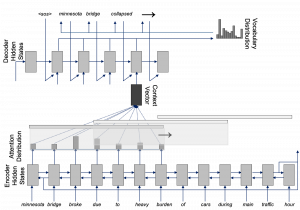The decoder uses the $$\rightarrow$$ to signal when it wants to move to the next window.

We construct the supervision via an unsupervised mapping heuristic between source and reference summary sentences. Each reference summary sentence $$S_{r_i}$$ is scored against every source sentence $$D_i$$ to determine the similarity between source and gold truth summary sentences. Two sentences are contrasted using cosine similarity, with

$$Sim(S_{r_i}, D_i) = \frac{a(S_{r_i}) \cdot a(D_i)}{\left|\left|a(S_{r_i})\right|\right| \cdot \left|\left|a(D_i)\right|\right|}$$,

where $$a(S_{r_i})$$ aggregates the word embeddings $$w_j \in S_{r_i}$$ by simple summation, i.e. $$a(S_{r_i}) = \sum_j w_j$$. Using these pseudo links between source and target summaries, we can reconstruct the hypothetical windows from which the writing of the ground truth sentences was most likely inspired by. Each target sentence receives a window number. Whenever a shift occurs, we insert the $$\rightarrow$$ symbol after the respective sentence in the ground truth summary.

Back to the example. We can see that we are now able to process the entire document. The model ejects $$\rightarrow$$ at some point, thereby signaling saturation of the first window. It then continues decoding the summary from the second window.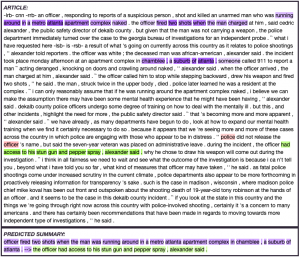Maximum attention visualization for windowing model

In an ultimate stress test, we apply our model to the Wikipedia entry about Lionel Messi with a document length of $$18,136$$ words which drastically exceeds the training boundary for the windowing model of $$T_x=1,160$$.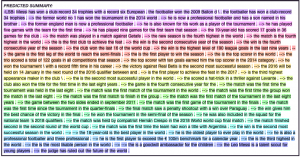Predicted summary for very long document (Wikipedia entry about Lionel Messi, extracted 2019/06/07) with windowing model

## Results measured by ROUGE on Validation Sets

SentenceGMODiseasesOrganic
"GMO food causes chronic diseases"0.450.450.1
"Organic food is healthy"0.10.10.8

The windowing models are often slightly worse compared to the standard model in terms of performance measured by ROUGE . For CNN/Dailymail, this is due to the information bias towards the first few sentences and the highly extractive nature of the summaries. That’s also why the LEAD-3 baseline achieves the best performance by simply taking the first three sentences of the document as summary. For WikiHow and in general, the standard model leads to higher scores due to its flexibility, as it can attend to the whole document at each decoding step, while a sliding windowing model has only access to a small content window. We would need an evaluation dataset with longer documents to prove the benefit of the windowing model in terms of performance. Alternatively, we can limit the setup for existing datasets: When comparing a windowing model (WIKI_II) that has $$\texttt{ws}=200$$ and is trained to slide to document lengths up to $$740$$ with a standard model (WIKI_I) that has only access to the first window, we can see that the windowing model clearly prevails.

## Conclusion

We succeed in extending recurrent Seq2Seq models for summarization of arbitrary long texts. The windowing model learns transitions on the dataset and is able to extrapolate with respect to document length on texts of any length during inference. As the standard model has more flexibility than it can attend over the entire document during training, we can prove a benefit measured by ROUGE only when restricting the length scope of the datasets.